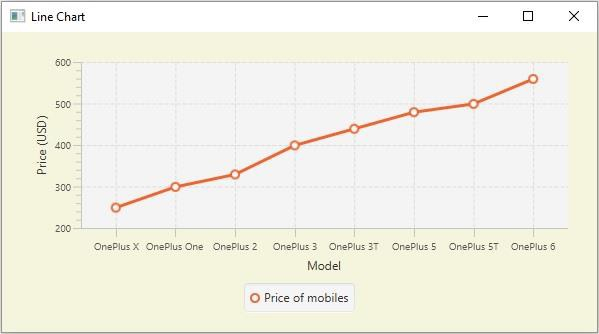# JavaFX LineChart example with category axis

Inline chart, the data values have represented a series of points connected by a line. In JavaFX, you can create a line chart by instantiating the javafx.scene.chart.LineChart class.

While instantiating this class you must pass the two objects of the Axis class representing the x and y-axis (as parameters of the constructor). Since the Axis class is abstract you need to pass objects of its concrete subclasses, NumberAxis (for numerical values) or, CategoryAxis (String values).

## Example

Following is an example demonstrating the usage of the category axis. Here, We are plotting the sales of various models of OnePlus mobile, we are using the Category (x) axis to plot the mobile models.

import javafx.application.Application;
import javafx.geometry.Insets;
import javafx.scene.Scene;
import javafx.stage.Stage;
import javafx.scene.chart.CategoryAxis;
import javafx.scene.chart.LineChart;
import javafx.scene.chart.NumberAxis;
import javafx.scene.chart.XYChart;
import javafx.scene.layout.StackPane;
public class LineChart_Category extends Application {
public void start(Stage stage) {
//Defining the x an y axes
CategoryAxis xAxis = new CategoryAxis();
NumberAxis yAxis = new NumberAxis(200, 600, 100);
//Setting labels for the axes
xAxis.setLabel("Model");
yAxis.setLabel("Price (USD)");
//Creating a line chart
LineChart linechart = new LineChart(xAxis, yAxis);
//Preparing the data points for the line
XYChart.Series series = new XYChart.Series();
//Setting the name to the line (series)
series.setName("Price of mobiles");
//Setting the data to Line chart
//Creating a stack pane to hold the chart
StackPane pane = new StackPane(linechart);
pane.setStyle("-fx-background-color: BEIGE");
//Setting the Scene
Scene scene = new Scene(pane, 595, 300);
stage.setTitle("Line Chart");
stage.setScene(scene);
stage.show();
}
public static void main(String args[]){
launch(args);
}
}

## Output